The goal of nethist is to estimate graphons by network histogram (Wolfe and Olhede 2013; Olhede and Wolfe 2014). It also provides extra tools for summary violin plot for networks (Maugis, Olhede, and Wolfe 2017) and visualizing network histogram. Use undirected and simple graphs with no self-loops (either igraph or matrix) as inputs for the functions in this package.

To install the package from source, you need C++ and Fortran compilers.

## Installation

You can install the development version of nethist from GitHub with:

# install.packages("devtools")
devtools::install_github("EnigmaSong/nethist")

## Example

Here are basic examples using political blog data set in the package:

### Network histogram

library(nethist)

We use polblog dataset in the package for our examples.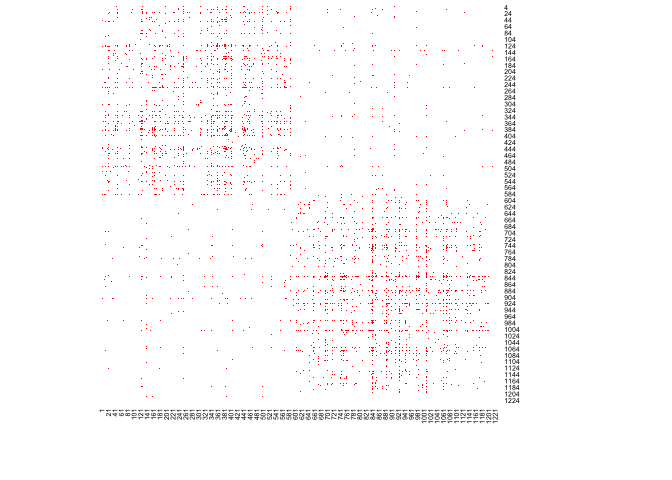We can estimate a network histogram from the political blog data and plot it.

## Example code using polblog data set
set.seed(42)
hist_polblog <- nethist(polblog, h = 72) #using user-specified bin size.
plot(hist_polblog)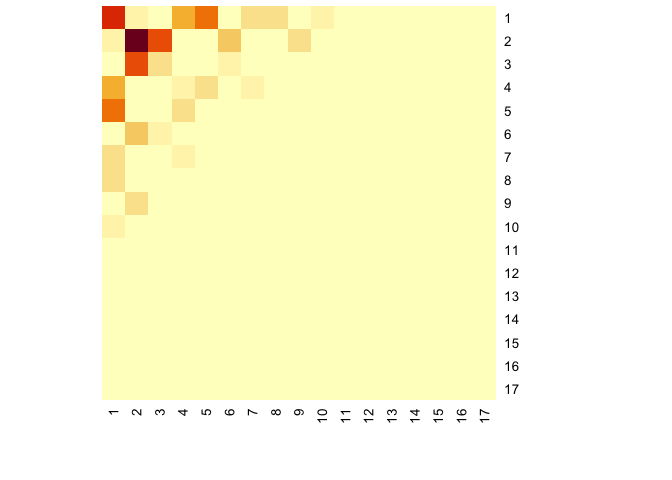### Plotting option

#### heatmap() style

plot() provides 2D plot as heatmap().

You can use a user-specified indices for plots. Here is an example:

print(ind)
#>   17 10 13  7 12  4  8  5  1  2  6  3  9 15 11 14 16
## Users can specify the index order of heatmap
plot(hist_polblog, idx_order = ind)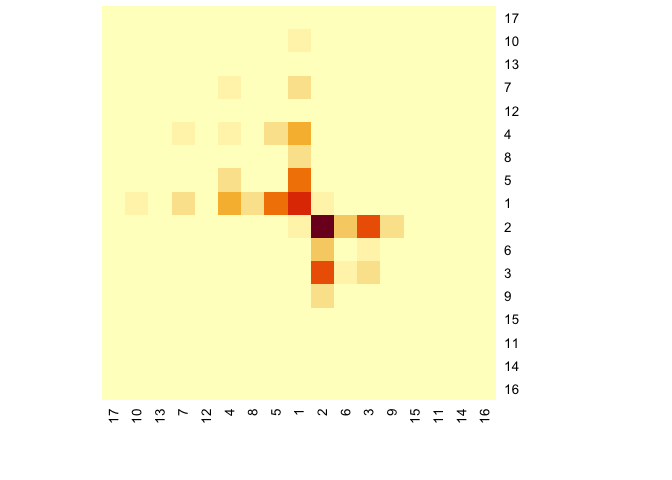## Users can specify the color palette
library(RColorBrewer)
plot(hist_polblog,  idx_order = ind, col = brewer.pal(9, "Greys"))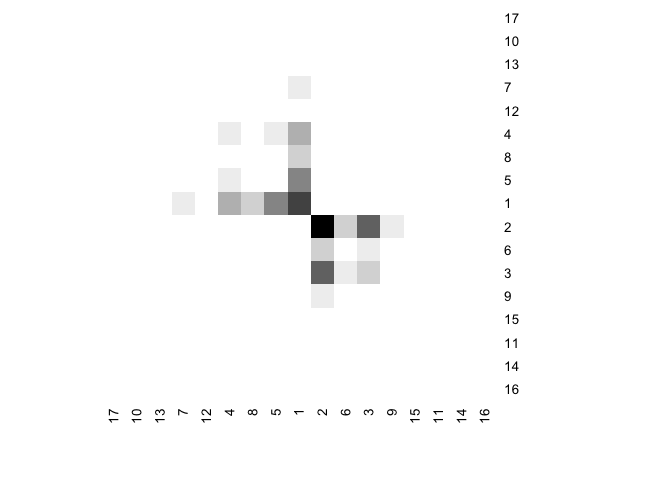You can display the estimated block probabilities by setting type = prob and prob=TRUE.

## Users can specify the color palette
plot(hist_polblog, idx_order = ind, type = "prob", prob= TRUE, prob.col = "blue",
col = colorRampPalette(colors=c("#FFFFFF","#000000"))(200))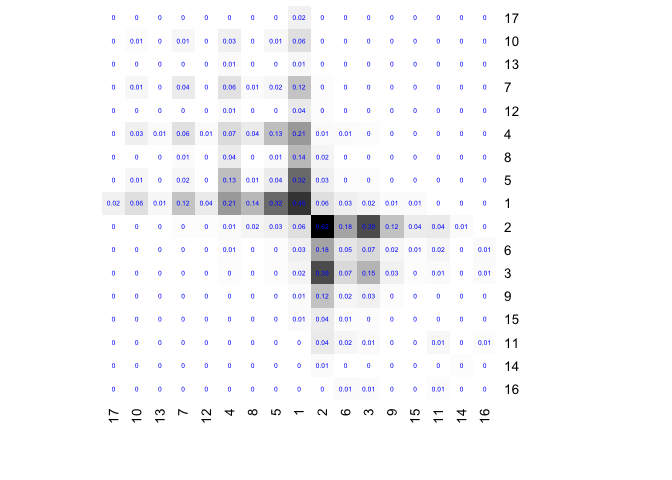### others

There are more types of plots in nethist package.

### Summary violin plot

If you want to check network summary violin plot of the data set:

#User-specified subsample size.
violin_netsummary(polblog, max_cycle_order = 7, subsample_sizes = 250)
#> Use R= 697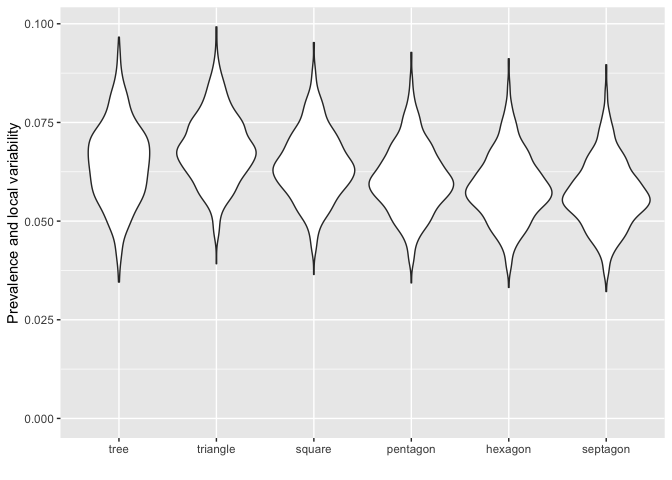#Auto-selected subsample size.
violin_netsummary(polblog, max_cycle_order = 7)
#> Use R= 697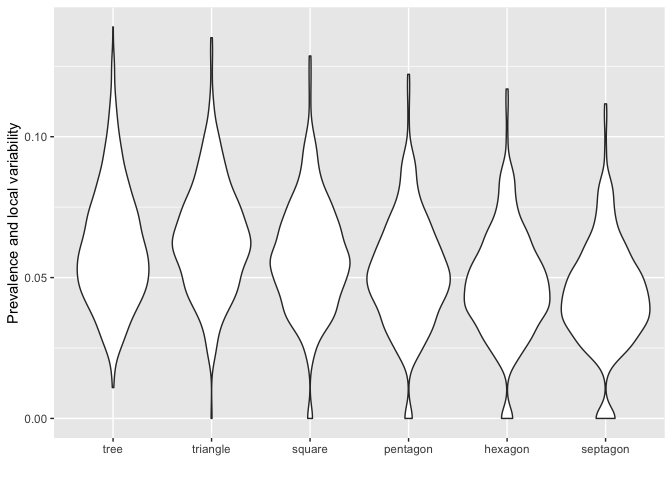## Reference

Maugis, Pierre-André G., Sofia C. Olhede, and Patrick J. Wolfe. 2017. “Topology Reveals Universal Features for Network Comparison.” arXiv:1705.05677, May. http://arxiv.org/abs/1705.05677.
Olhede, Sofia C., and Patrick J. Wolfe. 2014. “Network Histograms and Universality of Blockmodel Approximation.” Proceedings of the National Academy of Sciences 111 (41): 14722–27. https://doi.org/10.1073/pnas.1400374111.
Wolfe, Patrick J., and Sofia C. Olhede. 2013. “Nonparametric Graphon Estimation.” arXiv:1309.5936, September. http://arxiv.org/abs/1309.5936.